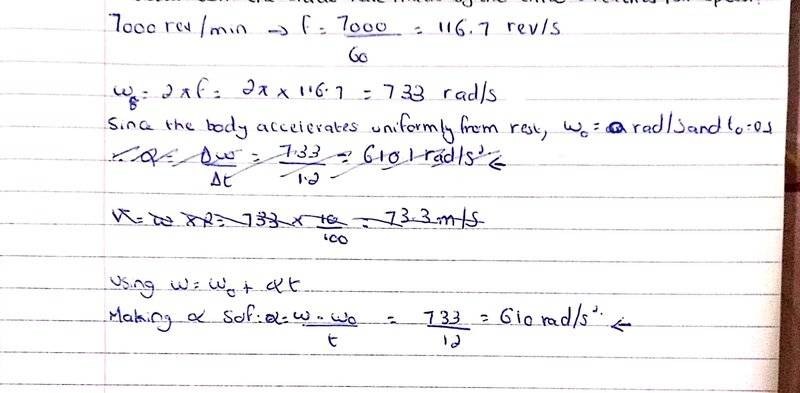# Motion with constant angular acceleration

Zoubayr
Homework Statement:
The blade of a circular saw of diameter 20 cm accelerates uniformly from rest to 7000 rev/min in 1.2 s. What is the angular acceleration? How many revolutions will the blade have made
by the time it reaches full speed?
Relevant Equations:
w=2πf
w=w_o +atLast edited by a moderator:

Mentor

I am not understanding the 2nd part of the question where it is asked about how many revolutions will the blade make when it reaches full speed.
The angular equations (position, velocity, acceleration) are analogous to their linear counterparts. For linear motion, what mathematical operation do you use (twice) to go from acceleration to distance traveled?Zoubayr
integration

•berkeman
Homework Helper
Gold Member
...